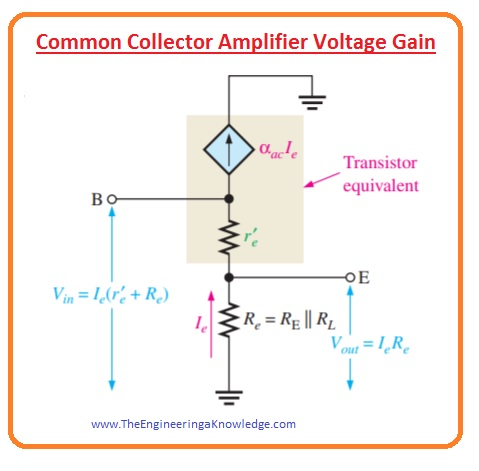Hello friends, I hope you all are doing great. In today’s tutorial, we will have a look at the Common collector Amplifier configuration and its applications. It is another category of BJT amplifier configuration in which input voltage is given at the base and output is taken at the emitter of the transistor. While collector is common between base and emitter terminals.

Such type of arrangement is known as a common collector because the collector is linked with the ground. In today’s post, we will have a detailed look at its circuit and some other related parameters. So let’s get started with Common Collector Amplifier.

#### Common Collector Amplifier

• You can see that input voltage is coupled with the base through the capacitor and collector is ground.
• The output signal is not inverted than the input signal but in same in magnitude to input.#### Common Collector Amplifier Voltage Gain

• As we know in all amplifiers the voltage gain is Av=Vout/Vin.
• The capacitive reactance will be ignored at frequency operation.
• In below figure ac model is shown.Vout=IeRe

Vin=Ie(r’e+Re)

• So the voltage gain equation will be.

Av=IeRe/Ie(r’e+Re)

• The base emitter gain expression will be as.

Av=Re/(r’e+Re)

• In this equation, Re is in parallel with the RE and RL. If a load is not connected then Re=RE.
• You can notice that gain is always less than the one.
• If Re>>r’e then the fine estimation will be.

Av=1

• As the output voltage is across the emitter it phase is similar to base voltage due to that there is no inversion occur from input to output.
• As there is no inversion at output as voltage gain is almost one the output voltage is the same as input for both phase and magnitude.

#### Common Collector Amplifier Input Resistance

• The input resistance for this common collector circuit is large.
• Due to large input resistance, it can be used as a buffer to reduce the loading effect. When the circuitry is running a load of less resistance.
• The calculation of input resistance observing from the base of the common-collector amplifier is like the common emitter amplifier which we discuss in the previous post.
• In common collector, though the emitter is never bypassed since the is taken about Re that is RE in parallel with the resistance RL.

Rin(base)=Vin/Iin=Vb/Ib

=Ie(r’e+Re)/Ib

As Ie=Ic=βacIb

Rin(base)=βacIb(r’e+Re)/Ib

• So we have after simplication.

Rin(base)=βac(r’e+Re)

• If Re>>r’e then the input resistance at base is after simplification.

Rin(base)=βacRe

• The bias resistance shown in the first figure is a parallel with the Rin(base) observing from the input like common emitter input resistance for a common collector is.

Rin(tot)=R1ІІR2ІІRin(base)

#### Output Resistance

• If load is not connected the output resistance will be given as.

Rout=(Rs/βac)ІІRE

• In this equation, Rs is the input resistance of the source

#### Current Gain

• The current for a common collector is given as.

Ai=Ie/Iin

• In this Iin is equal to the Vin/Rin(tot).

Power Gain

• The power gain is the product of current gain and voltage gain.
• For common-emitter power, the gain is one since voltage and current gain almost equal to each other.
• As Av=1 the power gain is

Ap=Ai

so, friends, it is a detailed post about the common collector amplifier if you have any post ask in comments. Thanks for reading. Have a good day.